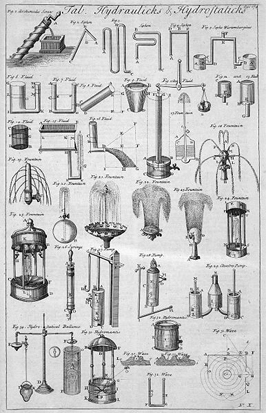Fluid staticsBy Wikipedia,
the free encyclopedia,

http://en.wikipedia.org/wiki/HydrostaticsTable of Hydraulics and Hydrostatics, from the 1728 Cyclopaedia

Fluid statics (also called hydrostatics) is the science of fluids at rest, and is a sub-field within fluid mechanics. The term usually refers to the mathematical treatment of the subject. It embraces the study of the conditions under which fluids are at rest in stable equilibrium. The use of fluid to do work is called hydraulics, and the science of fluids in motion is fluid dynamics.

## Pressure in fluids at rest

Due to the inability to resist deformation, fluids exert pressure normal to any contacting surface. In addition, when the fluid is at rest that pressure is isotropic, i.e. it acts with equal magnitude in all directions. This characteristic allows fluids to transmit force through the length of pipes or tubes, i.e., a force applied to a fluid in a pipe is transmitted, via the fluid, to the other end of the pipe. If the forces are not balanced, the fluid will move in the direction of the resulting force.

This concept was first formulated, in a slightly extended form, by the French mathematician and philosopher Blaise Pascal in 1647 and would later be known as Pascal's law. This law has many important applications in hydraulics.

### Hydrostatic pressure

In equilibrium, at every point in a fluid, the pressure is given by the weight per area of the column of fluid above it.

Consider a small cube of a liquid with uniform density ρ. The cube has edge length L and is located located at depth h. The force on this cube due to the weight of water above it has weight ρghL, where g denotes the gravitational constant. Because the system is in equilibrium, this force must be exactly balanced by pressure from the water on every other face of the cube. Thus, the pressure (force per area) at depth h given by$\ P = \rho g h$

where,

• P is the hydrostatic pressure (Pa);
• ρ is the liquid density (kg/m);
• g is gravitational acceleration (m/s);
• h is the height of liquid above (m).

This argument can be generalized to non-uniform fluids, giving$P=\int_h^0 \rho g s\,ds \,$

where the integral over the dummy variable s is from the depth in question to the location where pressure is defined to be zero (often, the liquid surface).

### Atmospheric pressure

Statistical mechanics shows that, for a gas of constant temperature, T, its pressure, p will vary with height, h, as:$\ p (h)=p (0) e^{-Mgh/kT}$

where:

g = the acceleration due to gravity
T = Absolute temperature (i.e. kelvins)
k = Boltzmann constant
M = mass of a single molecule of gas
p = pressure
h = height

If there are multiple types of molecules in the gas, the partial pressure of each type will be given by this equation. Under most conditions, the distribution of each species of gas is independent of the other species.

### Buoyancy

Any body of arbitrary shape which is immersed, partly or fully, in a fluid will experience the action of a net positive vertical force originating from the depth-dependent liquid pressure. This vertical force is termed buoyancy or buoyant force and is equal in magnitude, but opposite in direction, to the weight of the displaced fluid.

In the case of a ship, for instance, its weight is balanced by a buoyant force from the displaced water, allowing it to float. If more cargo is loaded onto the ship, it would sink more into the water - displacing more water and thus receive a higher buoyant force to balance the increased weight.

Discovery of the principle of buoyancy is attributed to Archimedes.

### Stability

A floating object is stable if it tends to restore itself to an equilibrium position after a small displacement. For example, floating objects will generally have vertical stability, as if the object is pushed down slightly, this will create a greater buoyant force, which, unbalanced against the weight force will push the object back up.

Rotational stability is of great importance to floating vessels. Given a small angular displacement, the vessel may return to its original position (stable), move away from its original position (unstable), or remain where it is (neutral).

Rotational stability depends on the relative lines of action of forces on an object. The upward buoyant force on an object acts through the centre of buoyancy, being the centroid of the displaced volume of fluid. The weight force on the object acts through its center of gravity. An object will be stable if an angular displacement moves the line of action of these forces to set up a 'righting moment'. See also Angle of loll.

## Liquids-fluids with free surfaces

Liquids can have free surfaces at which they interface with gases, or with a vacuum. In general, the lack of the ability to sustain a shear stress entails that free surfaces rapidly adjust towards an equilibrium. However, on small length scales, there is an important balancing force from surface tension.

### Surface tension effects

#### Capillary action

When liquids are constrained in vessels whose dimensions are small, compared to the relevant length scales, surface tension effects become important leading to the formation of a meniscus through capillary action. This capillary action has profound consequences for biological systems as it is part of one of the two driving mechanisms of the flow of water in plant xylem, the transpirational pull.

#### Drops

Without surface tension, drops would not be able to form. The dimensions and stability of drops are determined by surface tension.The drop's surface tension is directly proportional to the cohesion property of the fluid.

Text from Wikipedia is available under the Creative Commons Attribution/Share-Alike License; additional terms may apply.

Published - July 2009

Please see some ads intermixed with other content from this site:Copyright 2004-2021 © by Airports-Worldwide.com, Vyshenskoho st. 36, Lviv 79010, Ukraine
Legal Disclaimer